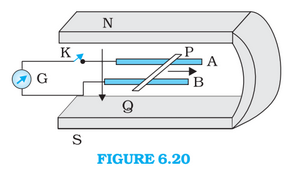# Q6.14 (a) Figure shows a metal rod   resting on the smooth rails  and positioned between the poles of a permanent magnet. The rails, the rod, and the magnetic field are in three mutual perpendicular directions. A galvanometer  connects the rails through a switch . Length of the ,  , resistance of the closed loop containing the . Assume the field to be uniform. Suppose K is open and the rod is moved with a speed of  in the direction shown. Give the polarity and magnitude of the induced emf.P Pankaj Sanodiya

Given

Length of the rod

Speed of the rod

Strength of the magnetic field

induced emf in the rod

Hence 9mV emf is induced and it is induced in a way such that P is positive and Q is negative.

Exams
Articles
Questions